Home Practice
For learners and parents For teachers and schools
Textbooks
Full catalogue
Pricing SupportLog in

We think you are located in United States. Is this correct?

# Chapter 5: Functions

• Learners must be able to determine the equation of a function from a given graph.
• Discuss and explain the characteristics of functions: domain, range, intercepts with the axes, maximum and minimum values, symmetry, etc.
• Emphasize to learners the importance of examining the equation of a function and anticipating the shape of the graph.
• Discuss the different functions and the effects of the parameters in general terms.

A function describes a specific relationship between two variables; where an independent (input) variable has exactly one dependent (output) variable. Every element in the domain maps to only one element in the range. Functions can be one-to-one relations or many-to-one relations. A many-to-one relation associates two or more values of the independent variable with a single value of the dependent variable. Functions allow us to visualise relationships in the form of graphs, which are much easier to read and interpret than lists of numbers.

### Revision (EMBGK)

#### Functions of the form $$y = a x^2 + q$$

Functions of the general form $$y=a{x}^{2}+q$$ are called parabolic functions, where $$a$$ and $$q$$ are constants.

The effects of $$a$$ and $$q$$ on $$f(x) = ax^2 + q$$:

• The effect of $$q$$ on vertical shift

• For $$q>0$$, $$f(x)$$ is shifted vertically upwards by $$q$$ units.

The turning point of $$f(x)$$ is above the $$x$$-axis.

• For $$q<0$$, $$f(x)$$ is shifted vertically downwards by $$q$$ units.

The turning point of $$f(x)$$ is below the $$x$$-axis.

• $$q$$ is also the $$y$$-intercept of the parabola.

• The effect of $$a$$ on shape

• For $$a>0$$; the graph of $$f(x)$$ is a “smile” and has a minimum turning point $$(0;q)$$. As the value of $$a$$ becomes larger, the graph becomes narrower.

As $$a$$ gets closer to $$\text{0}$$, $$f(x)$$ becomes wider.

• For $$a<0$$; the graph of $$f(x)$$ is a “frown” and has a maximum turning point $$(0;q)$$. As the value of $$a$$ becomes smaller, the graph becomes narrower.

As $$a$$ gets closer to $$\text{0}$$, $$f(x)$$ becomes wider.

 $$a<0$$ $$a>0$$ $$q>0$$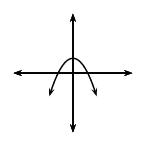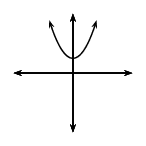$$q=0$$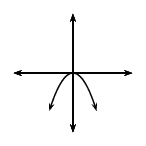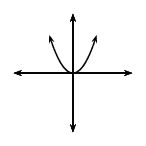$$q<0$$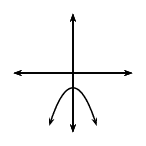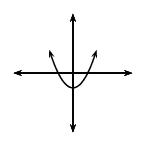## Revision

Textbook Exercise 5.1

On separate axes, accurately draw each of the following functions.

• Use tables of values if necessary.
• Use graph paper if available.

$$y_1 = x^2$$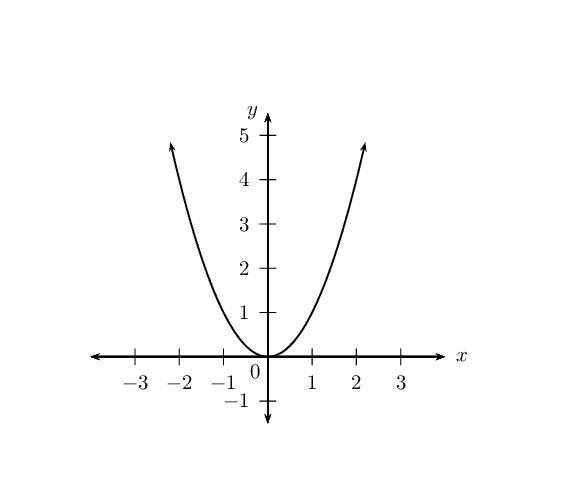$$y_2 = \frac{1}{2}x^2$$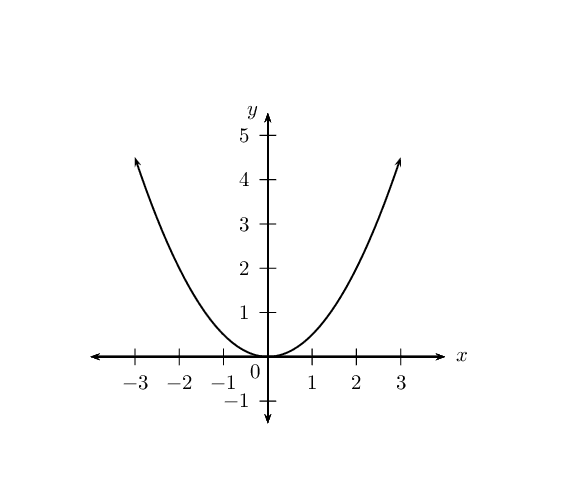$$y_3 = -x^2 - 1$$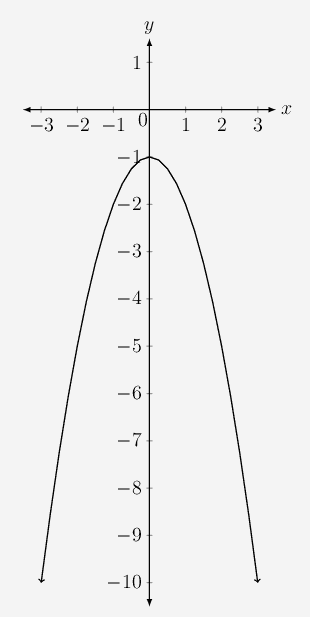$$y_4 = -2x^2 + 4$$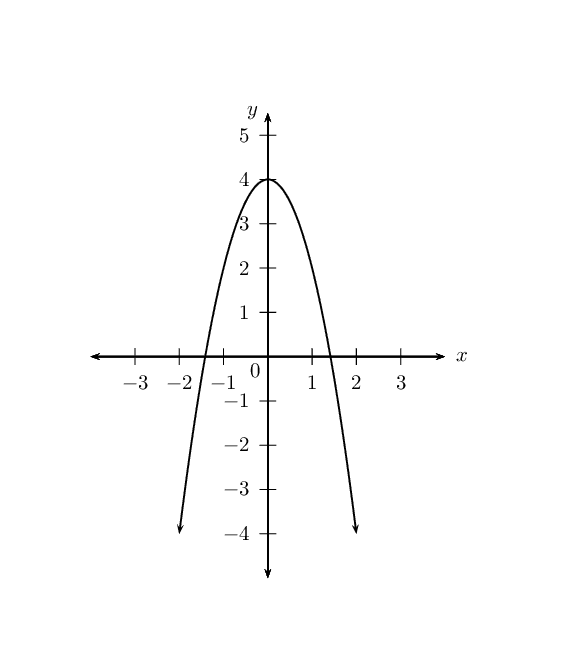Use your sketches of the functions given above to complete the following table (the first column has been completed as an example):

 $$y_1$$ $$y_2$$ $$y_3$$ $$y_4$$ value of $$q$$ $$q = 0$$ effect of $$q$$ $$y_{\text{int}} = 0$$ value of $$a$$ $$a = 1$$ effect of $$a$$ standard parabola turning point $$(0;0)$$ axis of symmetry $$x = 0$$ ($$y$$-axis) domain $$\{x: x \in \mathbb{R} \}$$ range $$\{y: y \geq 0\}$$
 $$y_1$$ $$y_2$$ $$y_3$$ $$y_4$$ value of $$q$$ $$q = \text{0}$$ $$q = \text{0}$$ $$q = -\text{1}$$ $$q = \text{4}$$ effect of $$q$$ $$y_{\text{int}} = 0$$ $$y_{\text{int}} = 0$$ $$y_{\text{int}} = -1$$, shifts $$\text{1}$$ unit down $$y_{\text{int}} = 4$$, shifts $$\text{4}$$ unit up value of $$a$$ $$a = 1$$ $$a = \frac{1}{2}$$ $$a = -1$$ $$a = -2$$ effect of $$a$$ standard parabola smile, min. TP frown, max. TP frown, max. TP turning point $$(0;0)$$ $$(0;0)$$ $$(0;-1)$$ $$(0;4)$$ axis of symmetry $$x = 0$$ ($$y$$-axis) $$x = 0$$ ($$y$$-axis) $$x = 0$$ ($$y$$-axis) $$x = 0$$ ($$y$$-axis) domain $$\{x: x \in \mathbb{R} \}$$ $$\{x: x \in \mathbb{R} \}$$ $$\{x: x \in \mathbb{R} \}$$ $$\{x: x \in \mathbb{R} \}$$ range $$\{y: y \geq 0\}$$ $$\{y: y \geq 0\}$$ $$\{y: y \leq -1\}$$ $$\{y: y \leq 4\}$$

### Functions of the form $$y=a{\left(x+p\right)}^{2}+q$$ (EMBGM)

We now consider parabolic functions of the form $$y=a{\left(x+p\right)}^{2}+q$$ and the effects of parameter $$p$$.

## The effects of $$a$$, $$p$$ and $$q$$ on a parabolic graph

1. On the same system of axes, plot the following graphs:

1. $$y_1 = x^2$$
2. $$y_2 = (x - 2)^2$$
3. $$y_3 = (x - 1)^2$$
4. $$y_4 = (x + 1)^2$$
5. $$y_5 = (x + 2)^2$$

Use your sketches of the functions above to complete the following table:

 $$y_1$$ $$y_2$$ $$y_3$$ $$y_4$$ $$y_5$$ $$x$$-intercept(s) $$y$$-intercept turning point axis of symmetry domain range effect of $$p$$
2. On the same system of axes, plot the following graphs:

1. $$y_1 = x^2 + 2$$
2. $$y_2 = (x - 2)^2 - 1$$
3. $$y_3 = (x - 1)^2 + 1$$
4. $$y_4 = (x + 1)^2 + 1$$
5. $$y_5 = (x + 2)^2 - 1$$

Use your sketches of the functions above to complete the following table:

 $$y_1$$ $$y_2$$ $$y_3$$ $$y_4$$ $$y_5$$ $$x$$-intercept(s) $$y$$-intercept turning point axis of symmetry domain range effect of $$q$$
3. Consider the three functions given below and answer the questions that follow:

• $$y_1 = (x - 2)^2 + 1$$
• $$y_2 = 2(x - 2)^2 + 1$$
• $$y_3 = -\frac{1}{2}(x - 2)^2 + 1$$
1. What is the value of $$a$$ for $$y_2$$?

2. Does $$y_1$$ have a minimum or maximum turning point?

3. What are the coordinates of the turning point of $$y_2$$?

4. Compare the graphs of $$y_1$$ and $$y_2$$. Discuss the similarities and differences.

5. What is the value of $$a$$ for $$y_3$$?

6. Will the graph of $$y_3$$ be narrower or wider than the graph of $$y_1$$?

7. Determine the coordinates of the turning point of $$y_3$$.

8. Compare the graphs of $$y_1$$ and $$y_3$$. Describe any differences.

The effect of the parameters on $$y = a(x + p)^2 + q$$

The effect of $$p$$ is a horizontal shift because all points are moved the same distance in the same direction (the entire graph slides to the left or to the right).

• For $$p>0$$, the graph is shifted to the left by $$p$$ units.

• For $$p<0$$, the graph is shifted to the right by $$p$$ units.

The value of $$p$$ also affects whether the turning point is to the left of the $$y$$-axis $$\left(p>0\right)$$ or to the right of the $$y$$-axis $$\left(p<0\right)$$. The axis of symmetry is the line $$x = -p$$.

The effect of $$q$$ is a vertical shift. The value of $$q$$ affects whether the turning point of the graph is above the $$x$$-axis $$\left(q>0\right)$$ or below the $$x$$-axis $$\left(q<0\right)$$.

The value of $$a$$ affects the shape of the graph. If $$a<0$$, the graph is a “frown” and has a maximum turning point. If $$a>0$$ then the graph is a “smile” and has a minimum turning point. When $$a = 0$$, the graph is a horizontal line $$y = q$$.

 $$p>0$$ $$p<0$$ $$a<0$$ $$a>0$$ $$a<0$$ $$a>0$$ $$q>0$$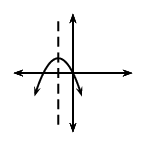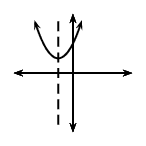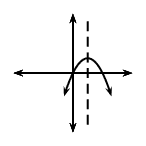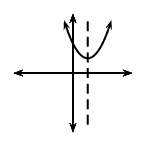$$q<0$$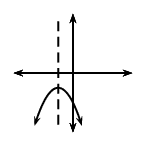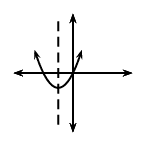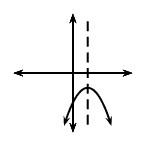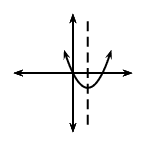#### Discovering the characteristics

For functions of the general form $$f(x) = y = a(x + p)^2 + q$$:

Domain and range

The domain is $$\left\{x:x\in ℝ\right\}$$ because there is no value of $$x$$ for which $$f(x)$$ is undefined.

The range of $$f(x)$$ depends on whether the value for $$a$$ is positive or negative. If $$a>0$$ we have: $\begin{array}{r@{\;}c@{\;}l@{\quad}l} (x + p)^2 & \geq & 0 & (\text{perfect square is always positive}) \\ \therefore a(x + p)^2 & \geq & 0 & (a \text{ is positive}) \\ \therefore a(x + p)^2 + q & \geq & q & \\ \therefore f(x) & \geq & q & \end{array}$

The range is therefore $$\{ y: y \geq q, y \in \mathbb{R} \}$$ if $$a > 0$$. Similarly, if $$a < 0$$, the range is $$\{ y: y \leq q, y \in \mathbb{R} \}$$.

## Worked example 1: Domain and range

State the domain and range for $$g(x) = -2(x - 1)^2 + 3$$.

### Determine the domain

The domain is $$\{x: x \in \mathbb{R} \}$$ because there is no value of $$x$$ for which $$g(x)$$ is undefined.

### Determine the range

The range of $$g(x)$$ can be calculated from: \begin{align*} (x - 1)^2& \geq 0 \\ -2(x - 1)^2& \leq 0 \\ -2(x - 1)^2 + 3 & \leq 3 \\ g(x) & \leq 3 \end{align*} Therefore the range is $$\{g(x): g(x) \leq 3 \}$$ or in interval notation $$(-\infty; 3]$$.

Notice in the example above that it helps to have the function in the form $$y = a(x + p)^2 + q$$.

We use the method of completing the square to write a quadratic function of the general form $$y = ax^2 + bx +c$$ in the form $$y = a(x + p)^2 + q$$ (see Chapter $$\text{2}$$).

## Domain and range

Textbook Exercise 5.2

Give the domain and range for each of the following functions:

$$f(x) = (x-4)^2 - 1$$

\begin{align*} \text{Domain: } & \left \{ x: x \in \mathbb{R} \right \} \\ \text{Range: } & \left \{ y: y \geq -1, y\in \mathbb{R} \right \} \end{align*}

$$g(x) = -(x-5)^2 + 4$$

\begin{align*} \text{Domain: } & \left \{ x: x \in \mathbb{R} \right \} \\ \text{Range: } & \left \{ y: y \leq 4, y\in \mathbb{R} \right \} \end{align*}

$$h(x) = x^2 -6x +9$$

\begin{align*} h(x) &= x^2 -6x +9 \\ &= (x - 3)^2 \\ \text{Domain: } & \left \{ x: x \in \mathbb{R} \right \} \\ \text{Range: } & \left \{ y: y \geq 0, y\in \mathbb{R} \right \} \end{align*}

$$j(x) = -2(x+1)^2$$

\begin{align*} \text{Domain: } & \left \{ x: x \in \mathbb{R} \right \} \\ \text{Range: } & \left \{ y: y \leq 0, y\in \mathbb{R} \right \} \end{align*}

$$k(x) = -x^2 + 2x - 3$$

\begin{align*} k(x) &= -x^2 + 2x - 3 \\ &= -(x - 1)^2 - 2 \\ \text{Domain: } & \left \{ x: x \in \mathbb{R} \right \} \\ \text{Range: } & \left \{ y: y \leq 2, y\in \mathbb{R} \right \} \end{align*}

Intercepts

The $$y$$-intercept:

Every point on the $$y$$-axis has an $$x$$-coordinate of $$\text{0}$$, therefore to calculate the $$y$$-intercept we let $$x=0$$.

For example, the $$y$$-intercept of $$g(x) = (x - 1)^2 + 5$$ is determined by setting $$x=0$$: \begin{align*} g(x) &= (x - 1)^2 + 5 \\ g(0) &= (0 - 1)^2 + 5 \\ &= 6 \end{align*} This gives the point $$(0;6)$$.

The $$x$$-intercept:

Every point on the $$x$$-axis has a $$y$$-coordinate of $$\text{0}$$, therefore to calculate the $$x$$-intercept we let $$y=0$$.

For example, the $$x$$-intercept of $$g(x) = (x - 1)^2 + 5$$ is determined by setting $$y=0$$: \begin{align*} g(x) &= (x - 1)^2 + 5 \\ 0 &= (x - 1)^2 + 5 \\ -5 &= (x - 1)^2 \end{align*} which has no real solutions. Therefore, the graph of $$g(x)$$ lies above the $$x$$-axis and does not have any $$x$$-intercepts.

## Intercepts

Textbook Exercise 5.3

Determine the $$x$$- and $$y$$-intercepts for each of the following functions:

$$f(x) = (x+4)^2 - 1$$

\begin{align*} \text{For } x=0 \quad y &= (0+4)^2 - 1 \\ &= 16 - 1 \\ \therefore & (0;15) \\ \text{For } y=0 \quad 0 &= (x+4)^2 - 1 \\ &= x^2 + 8x + 16 - 1 \\ &= x^2 + 8x + 15 \\ &= (x + 5)(x + 3) \\ x=-5 &\text{ or } x=-3 \\ \therefore (-5;0) &\text{ and } (-3;0) \end{align*}

$$g(x) = \text{16} - 8x + x^2$$

\begin{align*} \text{For } x=0 \quad y &=16 \\ \therefore & (0;16) \\ \text{For } y=0 \quad 0 &= 16 - 8x + x^2 \\ &= x^2 - 8x + 16 \\ &= (x -4)^2 \\ \therefore x &=4 \\ \therefore & (4;0) \end{align*}

$$h(x) = -x^2 +4x-3$$

\begin{align*} \text{For } x=0 \quad y &=-3 \\ \therefore & (0;-3) \\ \text{For } y=0 \quad 0 &= -x^2 +4x-3 \\ &= - (x^2 -4x+3) \\ &= -(x - 3)(x - 1) \\ x=3 &\text{ or } x=1 \\ \therefore (1;0) &\text{ and } (3;0) \end{align*}

$$j(x) = 4(x-3)^2 -1$$

\begin{align*} \text{For } x=0 \quad y &= 4(0-3)^2 -1 \\ &= 36 - 1 \\ \therefore & (0;35) \\ \text{For } y=0 \quad 0 &= 4(x-3)^2 -1 \\ &= 4(x^2 - 6x + 9) -1 \\ &= 4x^2 -24x + 36 - 1 \\ &= 4x^2 -36x + 35 \\ &= (2x + 5)(2x + 7) \\ x=-\frac{5}{2} &\text{ or } x=-\frac{7}{2} \\ \therefore (-\frac{5}{2};0) &\text{ and } (-\frac{7}{2};0) \end{align*}

$$k(x) = 4(x-3)^2 +1$$

\begin{align*} \text{For } x=0 \quad y &= 4(0-3)^2 +1 \\ &= 36 +1 \\ \therefore & (0;37) \\ \text{For } y=0 \quad 0 &= 4(x-3)^2 +1 \\ &= 4(x^2 - 6x + 9) +1 \\ &= 4x^2 -24x + 36 + 1 \\ &= 4x^2 -36x + 37 \\ \therefore x & = \frac{-b \pm \sqrt{b^2 - 4ac}}{2a} \\ & = \frac{24 \pm \sqrt{(-24)^2 - 4(4)(37)}}{2(4)} \\ & = \frac{576 \pm \sqrt{576 - 592}}{8} \\ & = \frac{576 \pm \sqrt{-16}}{8} \\ \therefore &\text{ no real solution } \end{align*}

$$l(x) = 2x^2 - 3x -4$$

\begin{align*} \text{For } x=0 \quad y &=-4 \\ \therefore & (0;-4) \\ \text{For } y=0 \quad 0 &= 2x^2 - 3x -4 \\ &= 2x^2 - 3x -4 \\ \therefore x & = \frac{-b \pm \sqrt{b^2 - 4ac}}{2a} \\ & = \frac{3 \pm \sqrt{(-3)^2 - 4(2)(-4)}}{2(2)} \\ & = \frac{3 \pm \sqrt{ 9 + 32}}{4} \\ & = \frac{3 \pm \sqrt{41}}{4} \\ x=\frac{3 - \sqrt{41}}{4} &\text{ or } x=\frac{3 + \sqrt{41}}{4} \\ \therefore (-\text{0,85};0) &\text{ and } (\text{2,35};0) \end{align*}

Turning point

The turning point of the function $$f(x) = a(x+p)^2 + q$$ is determined by examining the range of the function:

• If $$a > 0$$, $$f(x)$$ has a minimum turning point and the range is $$[q;\infty)$$:

The minimum value of $$f(x)$$ is $$q$$.

If $$f(x) = q$$, then $$a(x+p)^2 = 0$$, and therefore $$x = -p$$.

This gives the turning point $$(-p;q)$$.

• If $$a < 0$$, $$f(x)$$ has a maximum turning point and the range is $$(-\infty;q]$$:

The maximum value of $$f(x)$$ is $$q$$.

If $$f(x) = q$$, then $$a(x+p)^2 = 0$$, and therefore $$x = -p$$.

This gives the turning point $$(-p;q)$$.

Therefore the turning point of the quadratic function $$f(x) = a(x+p)^2 + q$$ is $$(-p;q)$$.

We can also write the quadratic equation in the form

$y = a(x - p)^2 +q$

The effect of $$p$$ is still a horizontal shift, however notice that:

• For $$p>0$$, the graph is shifted to the right by $$p$$ units.

• For $$p<0$$, the graph is shifted to the left by $$p$$ units.

The turning point is $$(p;q)$$ and the axis of symmetry is the line $$x = p$$.

## Worked example 2: Turning point

Determine the turning point of $$g(x) = 3x^2 - 6x - 1$$.

### Write the equation in the form $$y = a(x+p)^2 + q$$

We use the method of completing the square: \begin{align*} g(x )&= 3x^2 - 6x - 1 \\ &= 3(x^2 - 2x) - 1 \\ &= 3 \left( (x-1)^2 - 1 \right) -1 \\ &= 3(x-1)^2 - 3 -1 \\ &= 3(x-1)^2 - 4 \end{align*}

### Determine turning point $$(-p;q)$$

From the equation $$g(x) = 3(x-1)^2 - 4$$ we know that the turning point for $$g(x)$$ is $$(1;-4)$$.

## Worked example 3: Turning point

1. Show that the $$x$$-value for the turning point of $$h(x) = ax^2 + bx + c$$ is given by $$x = -\frac{b}{2a}$$.
2. Hence, determine the turning point of $$k(x) = 2 - 10x + 5x^2$$.

### Write the equation in the form $$y = a(x+p)^2 + q$$ and show that $$p = \frac{b}{2a}$$

We use the method of completing the square: \begin{align*} h(x)&= ax^2 + bx + c \\ &= a \left( x^2 + \frac{b}{a}x + \frac{c}{a} \right) \end{align*}

Take half the coefficient of the $$x$$ term and square it; then add and subtract it from the expression. \begin{align*} h(x) &= a \left( x^2 + \frac{b}{a}x + \left( \frac{b}{2a} \right)^2 - \left( \frac{b}{2a} \right)^2 + \frac{c}{a} \right) \\ &= a \left( \left(x + \frac{b}{2a} \right)^2 - \frac{b^2}{4a^2} + \frac{c}{a} \right) \\ &= a \left( \left(x + \frac{b}{2a} \right)^2 - \frac{b^2 -4ac}{4a^2} \right) \\ &= a \left(x + \frac{b}{2a} \right)^2 - \frac{b^2 -4ac}{4a} \end{align*} From the above we have that the turning point is at $$x = -p = - \frac{b}{2a}$$ and $$y = q = - \frac{b^2 -4ac}{4a}$$.

### Determine the turning point of $$k(x)$$

Write the equation in the general form $$y = ax^2 + bx + c$$. $k(x) = 5x^2 -10x + 2$ Therefore $$a = 5$$; $$b = -10$$; $$c = 2$$.

Use the results obtained above to determine $$x = - \frac{b}{2a}$$: \begin{align*} x &= -\left(\frac{-10}{2(5)}\right) \\ &= 1 \end{align*}

Substitute $$x = 1$$ to obtain the corresponding $$y$$-value: \begin{align*} y &= 5x^2 -10x + 2 \\ &= 5(1)^2 -10(1) + 2\\ &= 5 - \text{10} + 2\\ &= -3 \end{align*} The turning point of $$k(x)$$ is $$(1;-3)$$.

## Turning points

Textbook Exercise 5.4

Determine the turning point of each of the following:

$$y = x^2 - 6x + 8$$

\begin{align*} y &= x^2 - 6x + 8 \\ &= (x-3)^{2} - \left( \frac{6}{2} \right)^2 + 8 \\ &= (x-3)^{2}-1 \\ \therefore \text{turning point }&= (3;-1) \end{align*}

$$y = -x^2 + 4x - 3$$

\begin{align*} y &= -x^2 + 4x - 3 \\ &= -(x^2 - 4x + 3 \\ &= - \left( (x-2)^{2} - \left( \frac{4}{2} \right)^2 + 3 \right) \\ &= -(x-2)^{2}+1 \\ \therefore \text{turning point } &= (2;1) \end{align*}

$$y = \frac{1}{2}(x + 2)^2 - 1$$

\begin{align*} y &= \frac{1}{2}(x + 2)^2 - 1 \\ \therefore \text{turning point }&= (-2;-1) \end{align*}

$$y = 2x^2 + 2x + 1$$

\begin{align*} y &= 2x^2 + 2x + 1 \\ &= 2 \left( x^2 + x + \frac{1}{2} \right) \\ &= 2 \left( x+ \frac{1}{2} \right )^2+\frac{1}{2} \\ \therefore \text{turning point }&= \left(-\frac{1}{2};\frac{1}{2}\right) \end{align*}

$$y = \text{18} + 6x - 3x^2$$

\begin{align*} y &= -3x^2 + 6x + 18 \\ &= -3(x^2 - 2x - 6) \\ &= -3 \left((x - 1)^2 - 7 \right) \\ &= -3(x-1)^2+21 \\ \therefore \text{turning point }&= (1;21) \end{align*}

$$y = -2[(x + 1)^2 + 3]$$

\begin{align*} y &= -2[(x + 1)^2 + 3] \\ y &= -2(x + 1)^2 - 6 \\ \therefore \text{turning point }&= (-1;-6) \end{align*}

Axis of symmetry

The axis of symmetry for $$f(x)=a{\left(x+p\right)}^{2}+q$$ is the vertical line $$x=-p$$. The axis of symmetry passes through the turning point $$(-p;q)$$ and is parallel to the $$y$$-axis.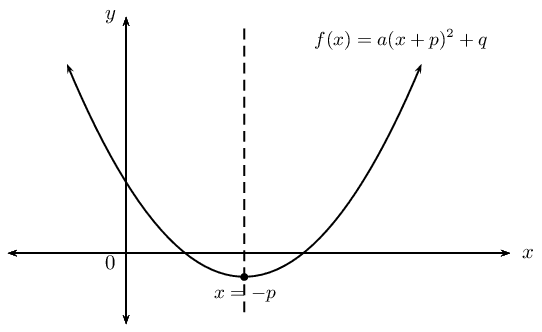## Axis of symmetry

Textbook Exercise 5.5

Determine the axis of symmetry of each of the following:

$$y = 2x^2 - 5x - \text{18}$$

\begin{align*} y &= 2x^2 - 5x - 18 \\ &= 2(x^2 - \frac{5}{2}x - 9) \\ &= 2\left( \left( x - \frac{5}{2} \right)^2 - \frac{25}{16} - 9 \right) \\ &= 2\left( \left( x - \frac{5}{2} \right)^2 - \frac{169}{16}\right) \\ &= 2\left( x - \frac{5}{4} \right)^2 - \frac{169}{8} \\ \text{Axis of symmetry: }x & =\frac{5}{4} \end{align*}

$$y = 3(x - 2)^2 + 1$$

\begin{align*} y &= 3(x - 2)^2 + 1 \\ \text{Axis of symmetry: } x & = 2 \end{align*}

$$y = 4x - x^2$$

\begin{align*} y &= 4x - x^2 \\ &= -(x^2 - 4x) \\ &= -(x - 2)^2 + 4 \\ \text{Axis of symmetry: } x & = 2 \end{align*}

Write down the equation of a parabola where the $$y$$-axis is the axis of symmetry.

$$y = ax^2 + q$$

#### Sketching graphs of the form $$f(x)=a{\left(x+p\right)}^{2}+q$$

In order to sketch graphs of the form $$f(x)=a{\left(x+p\right)}^{2}+q$$, we need to determine five characteristics:

• sign of $$a$$

• turning point

• $$y$$-intercept

• $$x$$-intercept(s) (if they exist)

• domain and range

## Worked example 4: Sketching a parabola

Sketch the graph of $$y = -\frac{1}{2}(x + 1)^2 - 3$$.

Mark the intercepts, turning point and the axis of symmetry. State the domain and range of the function.

### Examine the equation of the form $$y = a(x + p)^2 + q$$

We notice that $$a < 0$$, therefore the graph is a “frown” and has a maximum turning point.

### Determine the turning point $$(-p;q)$$

From the equation we know that the turning point is $$(-1; -3)$$.

### Determine the axis of symmetry $$x = -p$$

From the equation we know that the axis of symmetry is $$x = -1$$.

### Determine the $$y$$-intercept

The $$y$$-intercept is obtained by letting $$x = 0$$: \begin{align*} y &= -\frac{1}{2} \left((0) + 1 \right)^2 - 3\\ &= -\frac{1}{2} - 3\\ &= -3\frac{1}{2} \end{align*} This gives the point $$(0;-3\frac{1}{2})$$.

### Determine the $$x$$-intercepts

The $$x$$-intercepts are obtained by letting $$y = 0$$: \begin{align*} 0 &= -\frac{1}{2} \left(x + 1 \right)^2 - 3\\ 3 &= -\frac{1}{2} \left(x + 1 \right)^2 \\ -6 &= \left(x + 1 \right)^2 \end{align*} which has no real solutions. Therefore, there are no $$x$$-intercepts and the graph lies below the $$x$$-axis.

### Plot the points and sketch the graph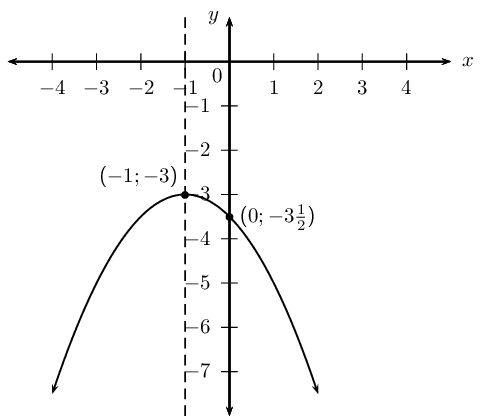### State the domain and range

Domain: $$\{ x: x \in \mathbb{R} \}$$

Range: $$\{ y: y \leq -3, y \in \mathbb{R} \}$$

## Worked example 5: Sketching a parabola

Sketch the graph of $$y = \frac{1}{2}x^2 - 4x + \frac{7}{2}$$.

Determine the intercepts, turning point and the axis of symmetry. Give the domain and range of the function.

### Examine the equation of the form $$y = ax^2 + bx +c$$

We notice that $$a > 0$$, therefore the graph is a “smile” and has a minimum turning point.

### Determine the turning point and the axis of symmetry

Check that the equation is in standard form and identify the coefficients.

$a = \frac{1}{2}; \qquad b = -4; \qquad c = \frac{7}{2}$

Calculate the $$x$$-value of the turning point using \begin{align*} x &= -\frac{b}{2a} \\ &= -\left(\frac{-4}{2\left( \frac{1}{2} \right)}\right) \\ &= 4 \end{align*} Therefore the axis of symmetry is $$x = 4$$.

Substitute $$x = 4$$ into the original equation to obtain the corresponding $$y$$-value. \begin{align*} y &= \frac{1}{2}x^2 - 4x + \frac{7}{2} \\ &= \frac{1}{2}(4)^2 - 4(4) + \frac{7}{2} \\ &= 8 -16 +\frac{7}{2} \\ &= -4\frac{1}{2} \end{align*} This gives the point $$\left( 4; -4\frac{1}{2} \right)$$.

### Determine the $$y$$-intercept

The $$y$$-intercept is obtained by letting $$x = 0$$: \begin{align*} y &= \frac{1}{2}(0)^2 - 4(0) + \frac{7}{2}\\ &= \frac{7}{2} \end{align*} This gives the point $$\left(0;\frac{7}{2}\right)$$.

### Determine the $$x$$-intercepts

The $$x$$-intercepts are obtained by letting $$y = 0$$: \begin{align*} 0 &= \frac{1}{2}x^2 - 4x + \frac{7}{2} \\ &= x^2 - 8x + 7 \\ &= (x - 1)(x - 7) \end{align*} Therefore $$x = 1$$ or $$x = 7$$. This gives the points $$(1;0)$$ and $$(7;0)$$.

### Plot the points and sketch the graph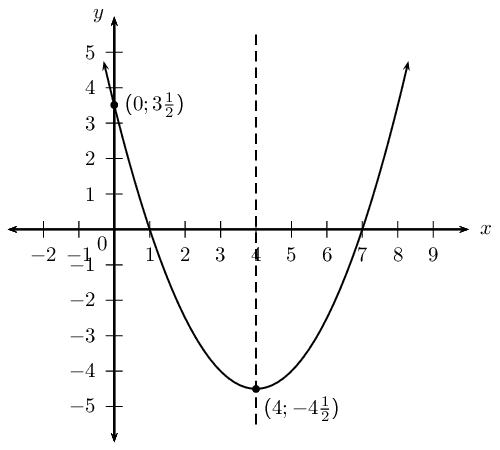### State the domain and range

Domain: $$\{ x: x \in \mathbb{R} \}$$

Range: $$\{ y: y \geq -4\frac{1}{2}, y \in \mathbb{R} \}$$

## Shifting the equation of a parabola

Carl and Eric are doing their Mathematics homework and decide to check each others answers.

Homework question:

If the parabola $$y = 3x^2 + 1$$ is shifted $$\text{2}$$ units to the right, determine the equation of the new parabola.

A shift to the right means moving in the positive $$x$$ direction, therefore $$x$$ is replaced with $$x + 2$$ and the new equation is $$y = 3(x + 2)^2 + 1$$.

We replace $$x$$ with $$x - 2$$, therefore the new equation is $$y = 3(x - 2)^2 + 1$$.

Work together in pairs. Discuss the two different answers and decide which one is correct. Use calculations and sketches to help explain your reasoning.

Writing an equation of a shifted parabola

The parabola is shifted horizontally:

• If the parabola is shifted $$m$$ units to the right, $$x$$ is replaced by $$(x-m)$$.

• If the parabola is shifted $$m$$ units to the left, $$x$$ is replaced by $$(x+m)$$.

The parabola is shifted vertically:

• If the parabola is shifted $$n$$ units down, $$y$$ is replaced by $$(y+n)$$.

• If the parabola is shifted $$n$$ units up, $$y$$ is replaced by $$(y-n)$$.

## Worked example 6: Shifting a parabola

Given $$y = x^2 - 2x -3$$.

1. If the parabola is shifted $$\text{1}$$ unit to the right, determine the new equation of the parabola.

2. If the parabola is shifted $$\text{3}$$ units down, determine the new equation of the parabola.

### Determine the new equation of the shifted parabola

1. The parabola is shifted $$\text{1}$$ unit to the right, so $$x$$ must be replaced by $$(x-1)$$. \begin{align*} y &= x^2 - 2x -3\\ &= (x-1)^2 - 2(x-1) -3 \\ &= x^2 - 2x + 1 -2x + 2 - 3\\ &= x^2 - 4x \end{align*} Be careful not to make a common error: replacing $$x$$ with $$x+1$$ for a shift to the right.

2. The parabola is shifted $$\text{3}$$ units down, so $$y$$ must be replaced by $$(y+3)$$. \begin{align*} y + 3&= x^2 - 2x -3\\ y &= x^2 - 2x -3 - 3 \\ &= x^2 - 2x -6 \end{align*}

## Sketching parabolas

Textbook Exercise 5.6

Sketch graphs of the following functions and determine:

• intercepts
• turning point
• axes of symmetry
• domain and range

$$y = -x^2 + 4x + 5$$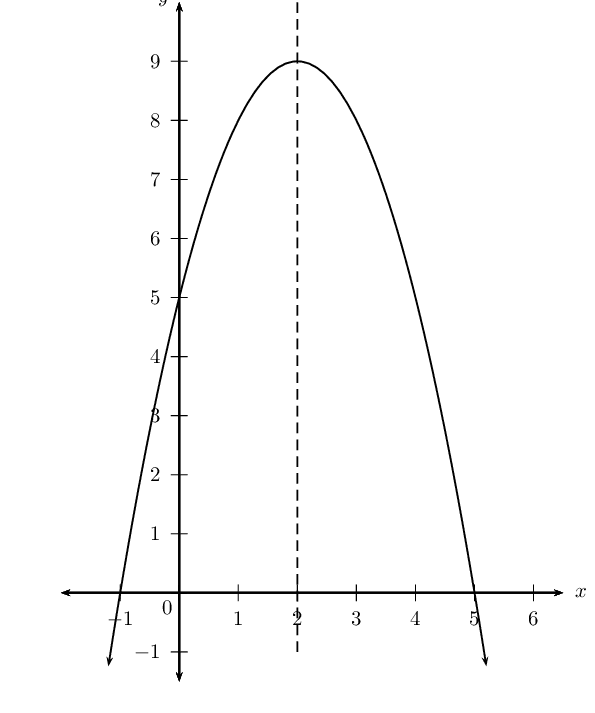$$y = 2(x + 1)^2$$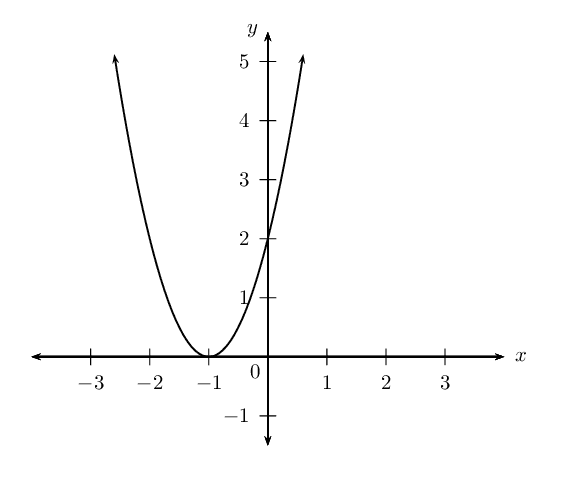$$y = 3x^2 - 2(x+2)$$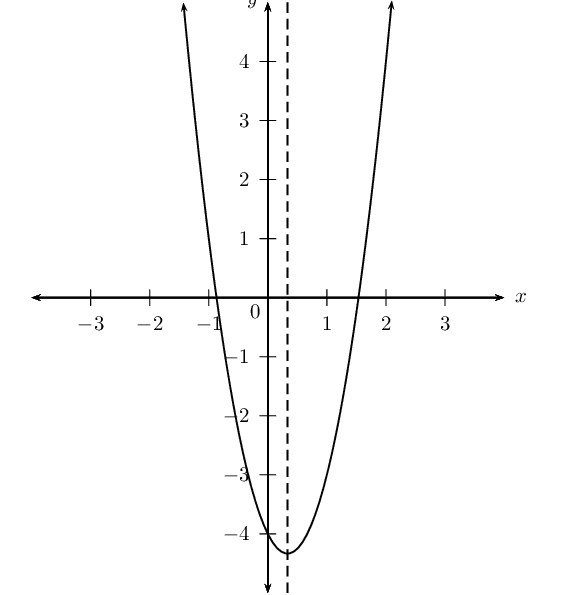$$y = 3(x -2)^2 + 1$$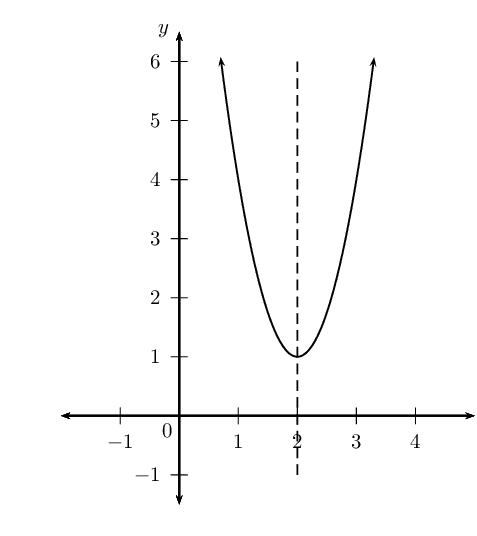Draw the following graphs on the same system of axes:

$$f(x) = -x^2 + 7$$

$$g(x) = -(x - 2)^2 + 7$$

$$h(x) = (x - 2)^2 - 7$$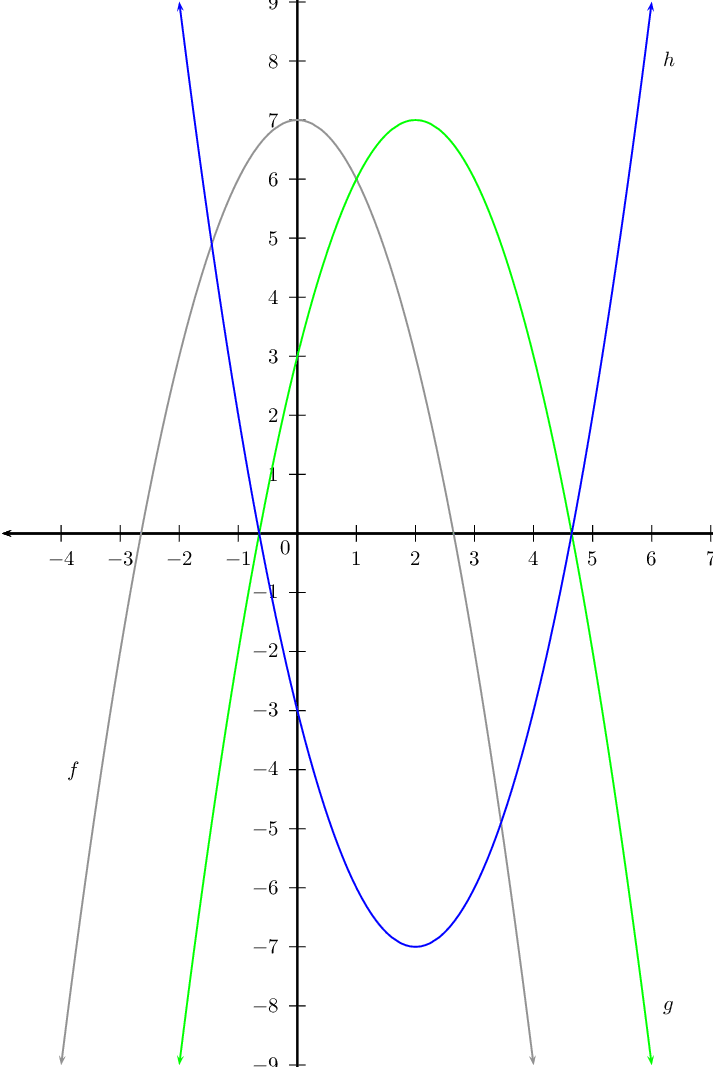Draw a sketch of each of the following graphs:

$$y = ax^2 + bx + c$$ if $$a > 0$$, $$b > 0$$, $$c < 0$$.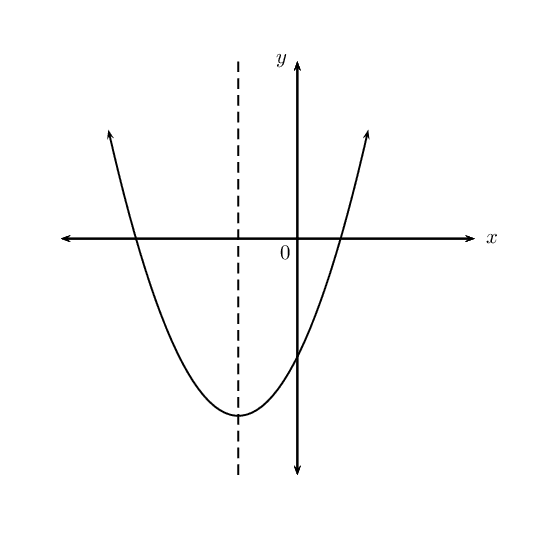$$y = ax^2 + bx + c$$ if $$a < 0$$, $$b = 0$$, $$c > 0$$.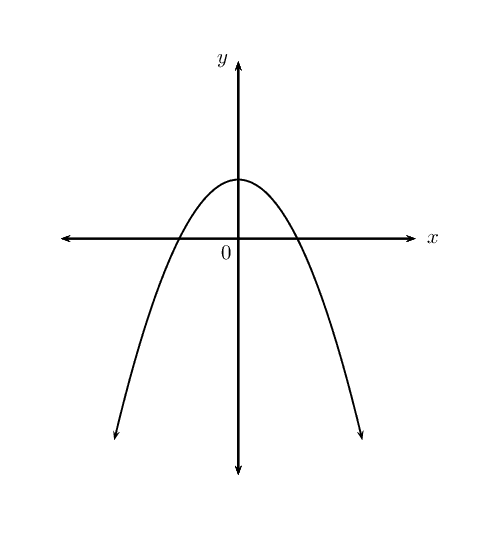$$y = ax^2 + bx + c$$ if $$a < 0$$, $$b < 0$$, $$b^2 - 4ac < 0$$.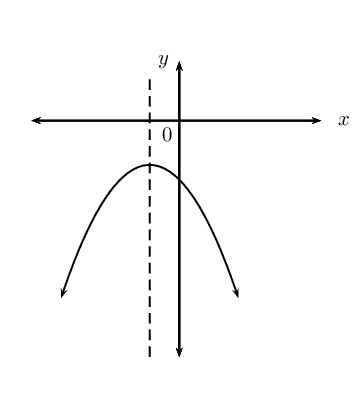$$y = (x+p)^2 + q$$ if $$p < 0$$, $$q < 0$$ and the $$x$$-intercepts have different signs.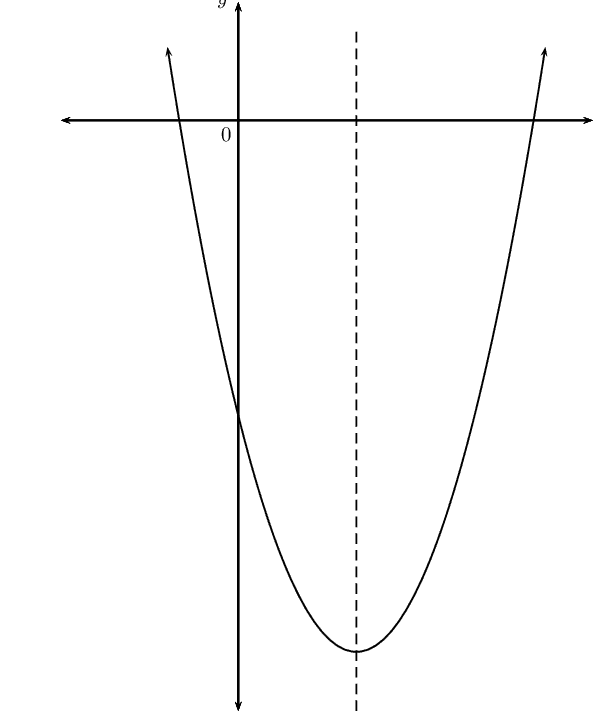$$y = a(x+p)^2 + q$$ if $$a < 0$$, $$p < 0$$, $$q > 0$$ and one root is zero.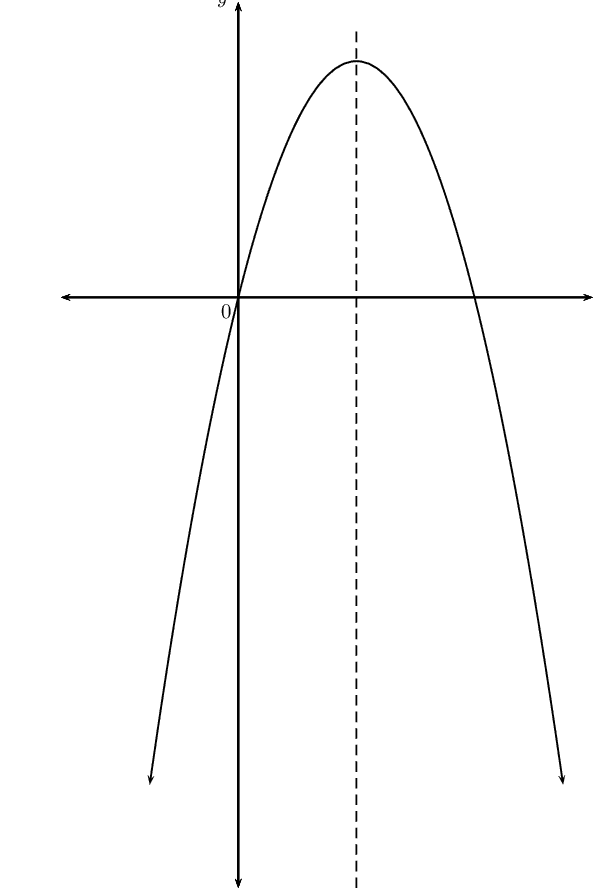$$y = a(x+p)^2 + q$$ if $$a > 0$$, $$p = 0$$, $$b^2 - 4ac > 0$$.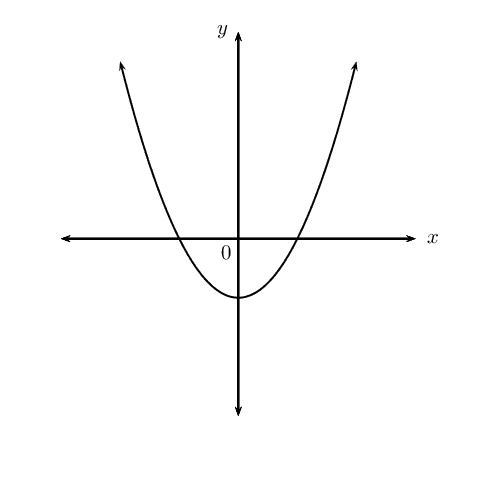Determine the new equation (in the form $$y = ax^2 + bx + c$$) if:

$$y = 2x^2 + 4x + 2$$ is shifted $$\text{3}$$ units to the left.

\begin{align*} y &=2x^2 + 4x + 2 \\ x &\Rightarrow x+3 \\ y_{\text{shifted}} &=2(x+3)^2 + 4(x+3) + 2 \\ &= 2(x^2 + 6x + 9) + 4x+12 + 2 \\ &= 2x^2 + 12x + 18 + 4x+12 + 2 \\ &= 2x^2 + 16x + 32 \end{align*}

$$y = -(x+1)^2$$ is shifted $$\text{1}$$ unit up.

\begin{align*} y &=2x^2 + 4x + 2 \\ y &\Rightarrow y-1 \\ y_{\text{shifted}} &= -(x+1)^2 + 1 \\ &= -(x^2 + 2x + 1) + 1 \\ &= -x^2 - 2x - 1 + 1 \\ &= -x^2 - 2x \end{align*}

$$y = 3(x-1)^2 + 2\left(x-\frac{1}{2}\right)$$ is shifted $$\text{2}$$ units to the right.

\begin{align*} y &= 3(x-1)^2 + 2\left(x-\frac{1}{2}\right) \\ x &\Rightarrow x-2 \\ y_{\text{shifted}} &= 3(x - 2-1)^2 + 2\left(x - 2 -\frac{1}{2}\right) \\ &= 3(x - 3)^2 + 2 \left(x - \frac{5}{2}\right) \\ &= 3(x^2 - 6x + 9) + 2x - 5 \\ &= 3x^2 - 18x + 27 + 2x - 5 \\ &= 3x^2 - 16x + 22 \end{align*}

Finding the equation of a parabola from the graph

If the intercepts are given, use $$y = a(x - x_1)(x - x_2)$$.

 Example: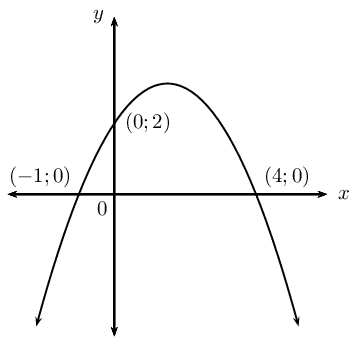$$x$$-intercepts: $$(-1;0)$$ and $$(4;0)$$ \begin{aligned} y &= a(x -x_1)(x - x_2) \\ &= a(x + 1)(x - 4) \\ &= ax^2 - 3ax - 4a \end{aligned} $$y$$-intercept: $$(0;2)$$ \begin{aligned} -4a &= 2 \\ a &= -\frac{1}{2} \end{aligned} Equation of the parabola: \begin{aligned} y &= ax^2 - 3ax - 4a \\ &= -\frac{1}{2}x^2 - 3\left(-\frac{1}{2}\right)x - 4\left(-\frac{1}{2}\right) \\ &= -\frac{1}{2}x^2 + \frac{3}{2}x + 2 \end{aligned}

If the $$x$$-intercepts and another point are given, use $$y = a(x -x_1)(x - x_2)$$.

 Example: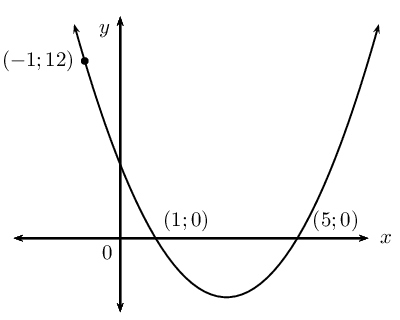$$x$$-intercepts: $$(1;0)$$ and $$(5;0)$$ \begin{aligned} y &= a(x -x_1)(x - x_2) \\ &= a(x - 1)(x - 5) \\ &= ax^2 - 6ax + 5a \end{aligned} Substitute the point: $$(-1;\text{12})$$ \begin{aligned} \text{12} &= a(-1)^2 - 6a(-1) + 5a \\ \text{12} &= a + 6a + 5a \\ \text{12} &= 12a \\ 1 &= a \end{aligned} Equation of the parabola: \begin{aligned} y &= ax^2 - 6ax + 5a \\ &= x^2 - 6x + 5 \end{aligned}

If the turning point and another point are given, use $$y = a(x + p)^2 + q$$.

 Example: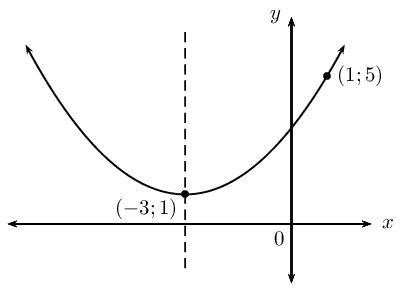Turning point: $$(-3;1)$$ \begin{aligned} y &= a(x + p)^2 + q \\ &= a(x + 3)^2 + 1 \\ &= ax^2 + 6ax + 9a + 1 \end{aligned} Substitute the point: $$(1;5)$$ \begin{aligned} 5 &= a(1)^2 + 6a(1) + 9a + 1 \\ 4 &= 16a \\ \frac{1}{4} &= a \end{aligned} Equation of the parabola: $$y = \frac{1}{4}(x + 3)^2 + 1$$

## Finding the equation

Textbook Exercise 5.7

Determine the equations of the following graphs. Write your answers in the form $$y = a(x + p)^2 + q$$.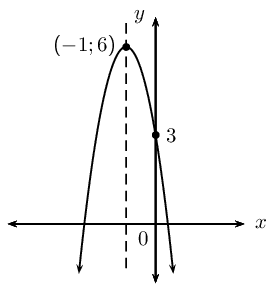\begin{align*} y &= a(x + p)^2 + q \\ \text{Subst. } & (-1;6) \\ y &=a(x+1)^2+6 \\ &=ax^2+2ax+a+6 \\ y-\text{int: } &= (0;3) \\ 3 &=0 +0 +a+6 \\ \therefore 3 &= a + 6 \\ a &= -3 \\ y=-3(x+1)^2+6 &\text{ or } y =-3x^2-6x+3 \end{align*}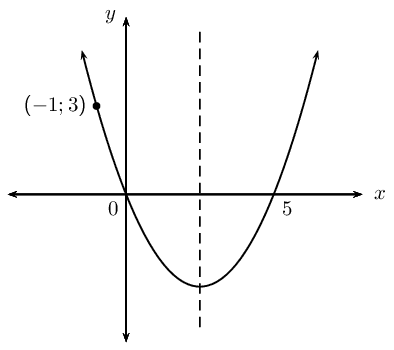\begin{align*} y &= a(x + p)^2 + q \\ y&=a(x-0)(x-5) \\ &=ax^2-5ax \\ \text{Subst. } & (-1;3) \\ y &=ax^2-5ax \\ 3 &=a(-1)^2-5a(-1) \\ 3 &= a+5a \\ 3 &= 6a \\ \therefore a &= \frac{1}{2} \\ \therefore y&=\frac{1}{2}x^2-\frac{5}{2}x \end{align*}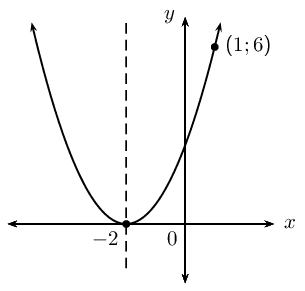\begin{align*} y &= a(x + p)^2 + q \\ \text{Subst. } &= (-2;0) \\ y &= a(x + 2)^2 \\ &=ax^2+4ax+4a \\ \text{Subst. } & (1;6) \\ 6 &= a +4a +4a \\ 6 &=9a \\ \therefore a &=\frac{2}{3} \\ \therefore y &= \frac{2}{3}(x+2)^2 \end{align*}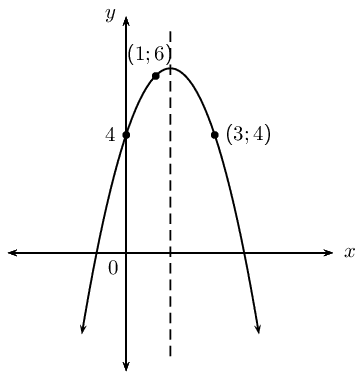\begin{align*} y &= ax^2+bx+c \\ y &=ax^2+bx+4 \\ \text{Subst. }(1;6): \qquad 6&=a+b+4 \ldots (1) \\ \text{Eqn. }(1) \times 5: \qquad 30 &=5a+5b+20 \ldots (3) \\ \text{Subst. }(1;6): \qquad -6&=25a+5b+4 \ldots (2) \\ (2) - (3) \quad -36&=20a-16 \\ 20a&=-20 \\ a &= -1 \\ b&=3 \\ c&= 4 \\ \therefore y&=-x^2+3x+4 \end{align*}
temp text